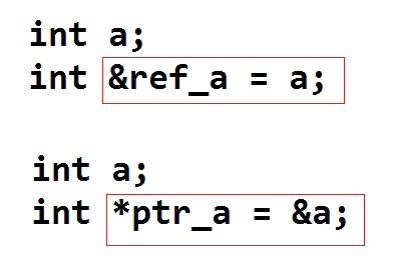# Advantages of reference variable over pointer variable in C++Reference variables are the alias of another variable while pointer variable are the special type of variable that contains the address of another variable.

Reference and pointers both can be used to refer the actual variable they provide the direct access to the variable.

But, references have some advantages over the pointer variables, those are:

In pointers - To access the value of actual variable, we need to explicitly deference the pointer variable by using ‘value at address’ operator/ dereferencing operator (*).

In references - To access the value of actual variable, we do not need to explicitly dereference the reference variable, they gets de-referenced automatically.

Reference variables are cleaner and modish as compare to the pointers; they can also be used while passing in the function as arguments, known as call by references.

Here is an example of reference variable in C++

```#include <iostream>
using namespace std;

int main()
{
int a=10;
int &ref_a=a;

cout<<"a: "<<a<<", ref_a: "<<ref_a<<endl;
ref_a=100;
cout<<"a: "<<a<<", ref_a: "<<ref_a<<endl;

return 0;
}
```

Output

```a: 10, ref_a: 10
a: 100, ref_a: 100
```

Here, ref_a is the reference variable of a, we can use it anywhere to access, edit the value of a, in this program we are accessing and changing the value of a through ref_a.

Languages: » C » C++ » C++ STL » Java » Data Structure » C#.Net » Android » Kotlin » SQL
Web Technologies: » PHP » Python » JavaScript » CSS » Ajax » Node.js » Web programming/HTML
Solved programs: » C » C++ » DS » Java » C#
Aptitude que. & ans.: » C » C++ » Java » DBMS
Interview que. & ans.: » C » Embedded C » Java » SEO » HR
CS Subjects: » CS Basics » O.S. » Networks » DBMS » Embedded Systems » Cloud Computing
» Machine learning » CS Organizations » Linux » DOS
More: » Articles » Puzzles » News/Updates